# The entropy change for NH,(9) + NH3(aq) will probably be: Select the correct answer below: Negative...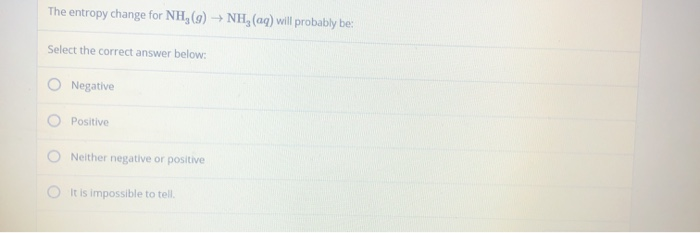The entropy change for NH,(9) + NH3(aq) will probably be: Select the correct answer below: Negative Positive Neither negative or positive It is impossible to tell

Please give me thumbs up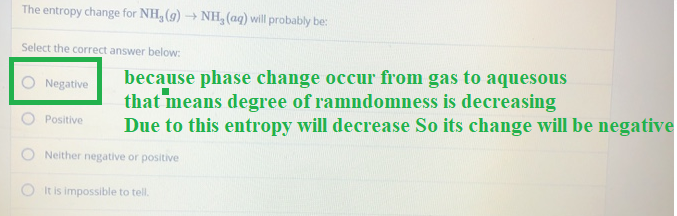##### Add Answer of: The entropy change for NH,(9) + NH3(aq) will probably be: Select the correct answer below: Negative...
Similar Homework Help Questions
• ### Consider the reaction: NH CH3) NH3(g) + HCl(aq) Using standard absolute entropies at 298K, calculate the...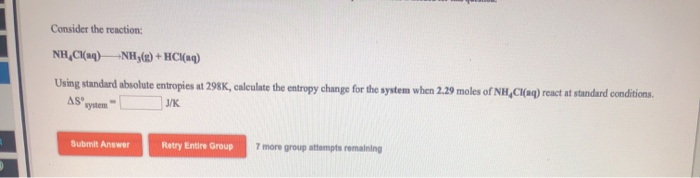Consider the reaction: NH CH3) NH3(g) + HCl(aq) Using standard absolute entropies at 298K, calculate the entropy change for the system when 2.29 moles of NH Cl(aq) react at standard conditions. Asystem / Submit Answer Retry Entire Group 7 more group attempts remaining

• ### Select all of the answers below that would lead to a spontaneous process. Group of answer...

Select all of the answers below that would lead to a spontaneous process. Group of answer choices a negative change in the entropy of the universe a positive change in the entropy of the universe a positive change in the entropy of the system with a magnitude that is smaller than a negative change in the entropy of the surroundings a positive change in the entropy of the system with a magnitude that is greater than a negative change in...

• ### Identify types of reactions Involving acids and bases Question In the reaction H3PO4(aq) +3 NH3(aq) 3...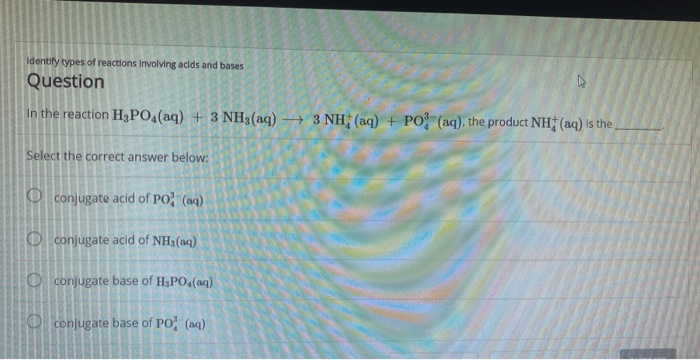Identify types of reactions Involving acids and bases Question In the reaction H3PO4(aq) +3 NH3(aq) 3 NH (aq) + PO (aq), the product NH (aq) is the Select the correct answer below: Oconjugate acid of PO (aq) Oconjugate acid of NHa(aq) Oconjugate base of H,PO4(aq) Oconjugate base of PO (aq)

• ### Question 7 Predict change in entropy for H2 + O2 --> 2H20 (1 point) negative none...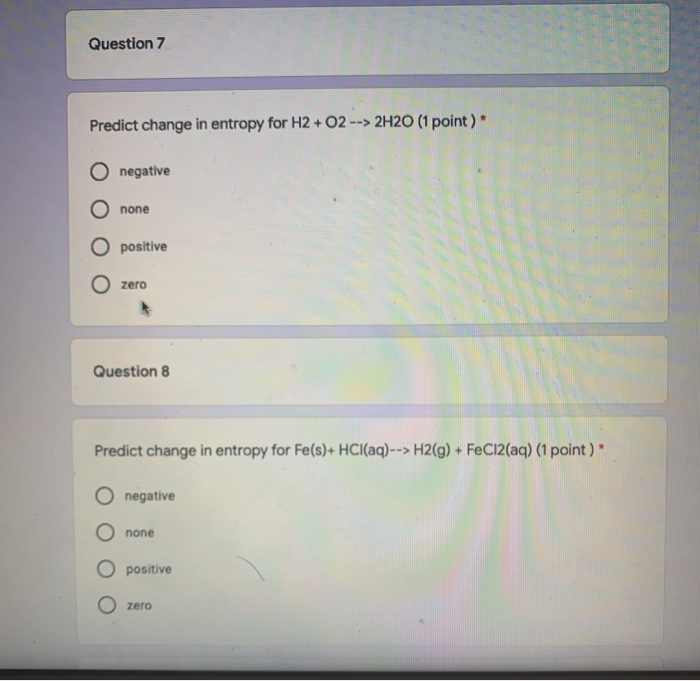Question 7 Predict change in entropy for H2 + O2 --> 2H20 (1 point) negative none O positive O zero Question 8 Predict change in entropy for Fe(s)+ HCl(aq)--> H2(g) + FeCl2(aq) (1 point)* negative none positive zero Show transcribed image text

• ### 4. Be sure to answer all parts. Predict whether the entropy change is positive or negative...

4. Be sure to answer all parts. Predict whether the entropy change is positive or negative for each of the following reactions KCIO6) 2 KCIO()+0.2) O positive O negative positive O negative

• ### Short Answer: (4 points each) 1.) Predict whether the entropy change is positive or negative for...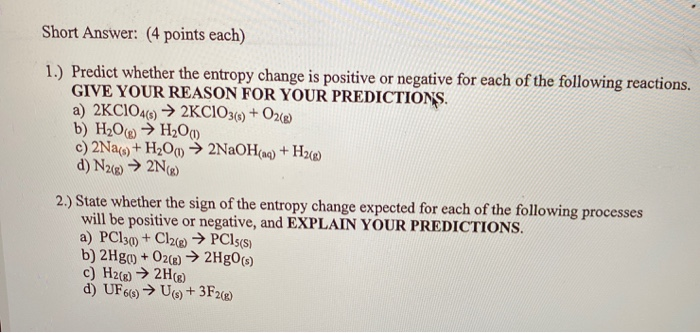Short Answer: (4 points each) 1.) Predict whether the entropy change is positive or negative for each of the following reactions. GIVE YOUR REASON FOR YOUR PREDICTIONS a) 2KC1O4(s) → 2KCIO3() + O2(8) b) H2O → H2O) c) 2Na(3) + H2O → 2NaOH(aq) + H2() d) N2(g) → 2N) 2.) State whether the sign of the entropy change expected for each of the following processes will be positive or negative, and EXPLAIN YOUR PREDICTIONS. a) PC130) + Cl2(e) → PCls(s)...

• ### Determine the standard entropy change of the universe when 0.2 mol of NH,NO3 is produced, NH4+...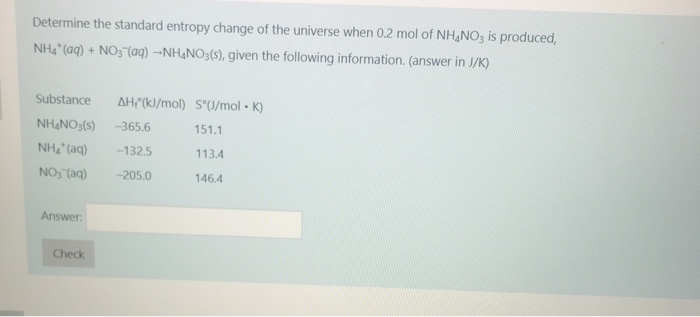Determine the standard entropy change of the universe when 0.2 mol of NH,NO3 is produced, NH4+ (aq) + NO3-(aq) --NH.NO3(s), given the following information. (answer in J/K) Substance NH.NO3(s) AH!"(kJ/mol) SºU/molK) -365.6 151.1 -132.5 113.4 -205.0 146.4 NH" (G) NO3(aq) Answer: Check

• ### A scientist measures the standard enthaply change for the following reaction to be 80.4kJ NH4Cl(aq)=NH3(g)+HCl(aq) B...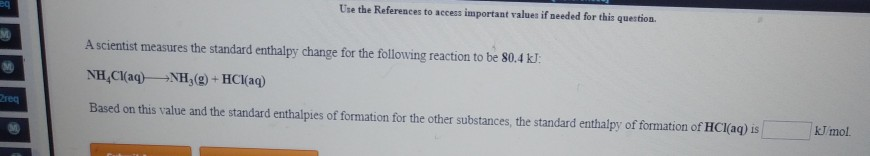A scientist measures the standard enthaply change for the following reaction to be 80.4kJ NH4Cl(aq)=NH3(g)+HCl(aq) Based on this value and the standard enthalpies of formation for the other substances, the standard enthaply of formation of HCl (aq) Use the References to access important values if needed for this question A scientist measures the standard enthalpy change for the following reaction to be 80.4 kJ. NH Cl(aq) +NH (9) + HCl(aq) G Based on this value and the standard enthalpies of...

• ### 3) Predict whether the change in entropy is positive, negative, or zero for each of the...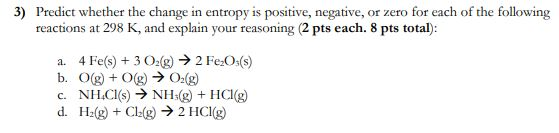3) Predict whether the change in entropy is positive, negative, or zero for each of the following reactions at 298 K, and explain your reasoning (2 pts each. 8 pts total): 1.4 Fe(s) + 3 O2(g) → 2 Fe:O:(s) b. O(g) + ( ) → O.(g) NH,Cl(s) » NH(g) + HCl(g) d. Hz(g) + Cl:(g) → 2 HCI c.

• ### Predict sign of Change in EntropyPredict, using the intuitive ideas about entropy,whether Ssys will bepositive, negative, or essentiallyzero for each of the following:a.Ca(OH)2(s) + CO2(g) CaCO3(s) +H2O(g)b.CuSO4(s) Cu2+(aq)+SO42-(aq)c.2HCl(g) + Br2(l) 2HBr(g) + Cl2(g)d.SO2(g) + 1/2 O2(g) SO3(g)e.Cu2+(aq) + 4NH3(aq) Cu(NH3)42+(aq)I used the equationn(gas)=(sum of coefficients of gases in theproducts)-(sum ofcoefficients of gases in the reactants). This is what Ifound:a.1-1=0b.0-0=0c.3-2=1d.1-1.5= -0.5e.0-0=0-The sign of Scorresponds to the sign of n. Therefore, I know C must have a positive Sand D must have a negative S.-Neither B nor...

Free Homework App